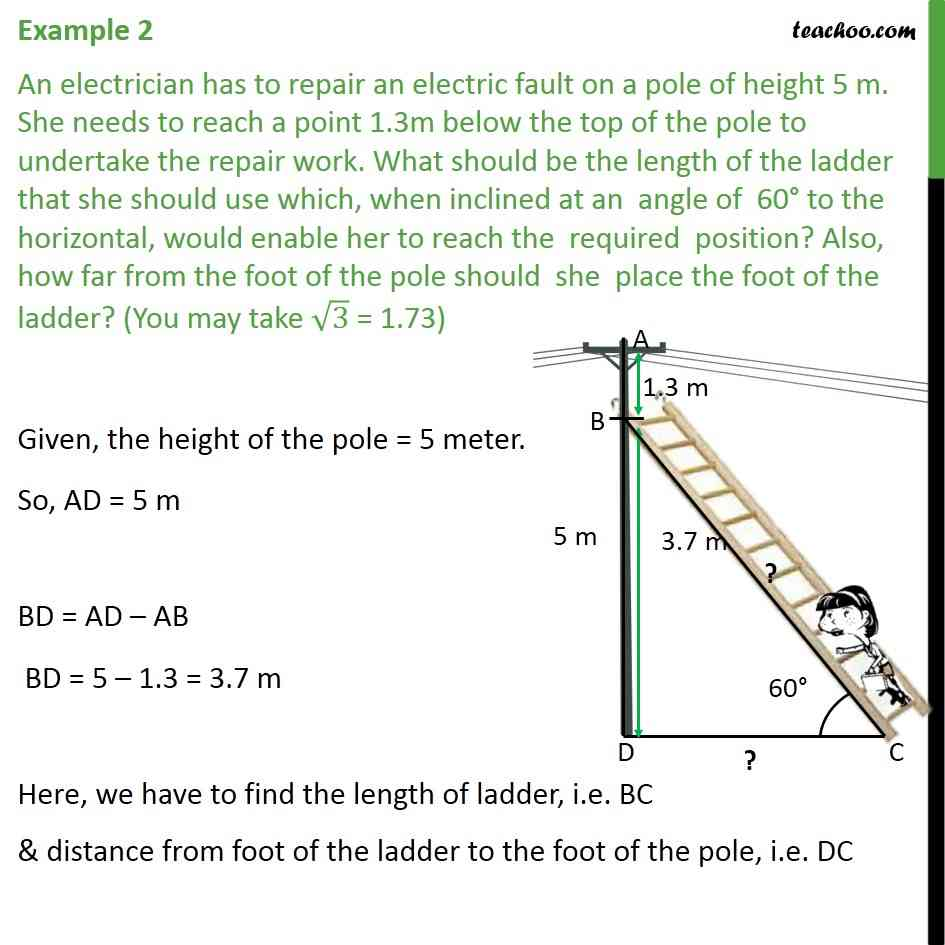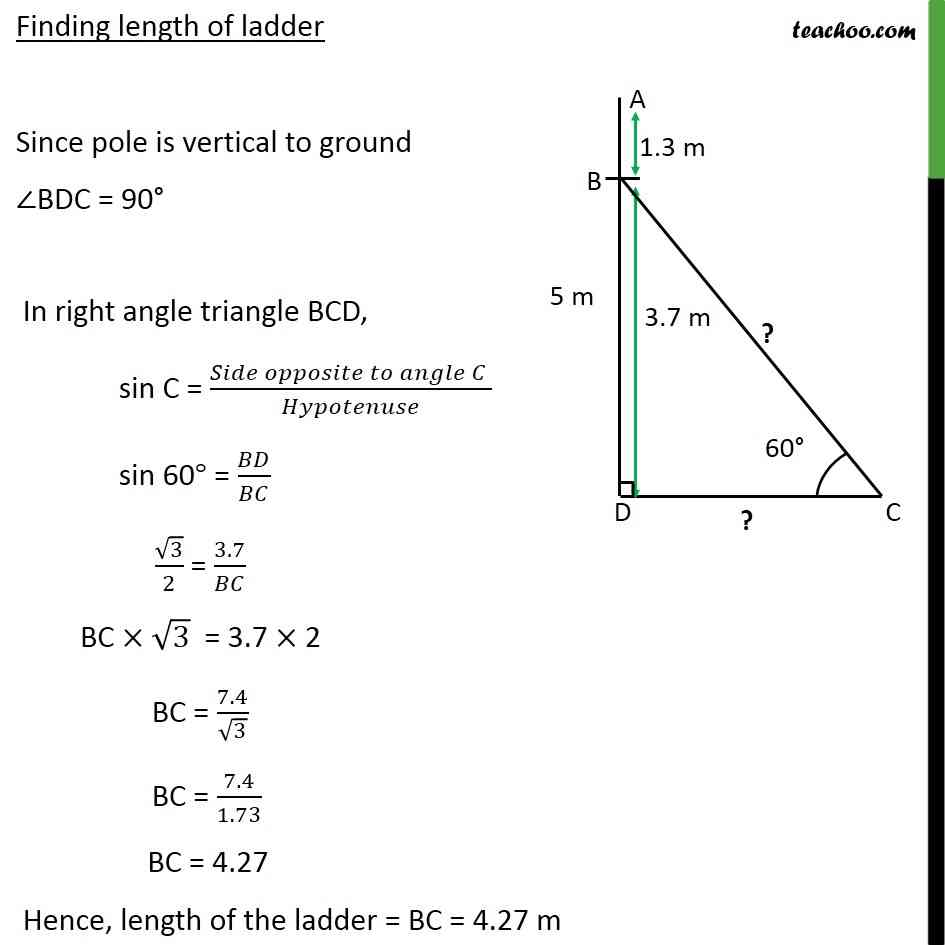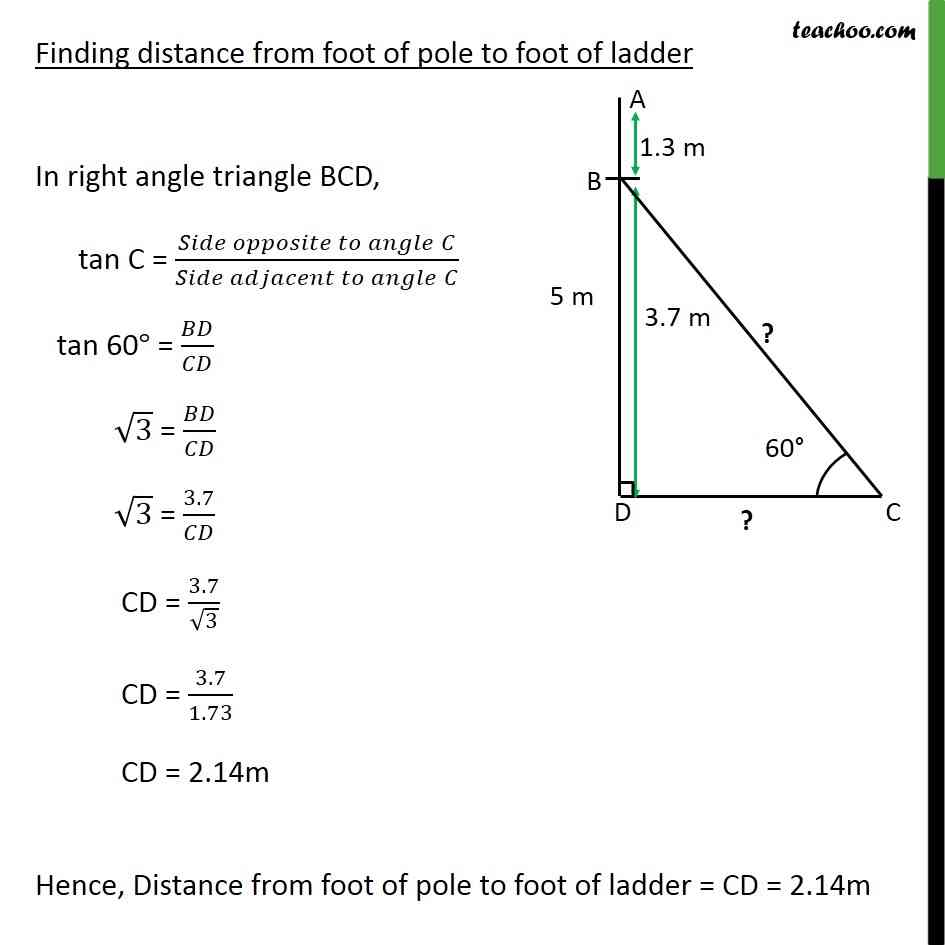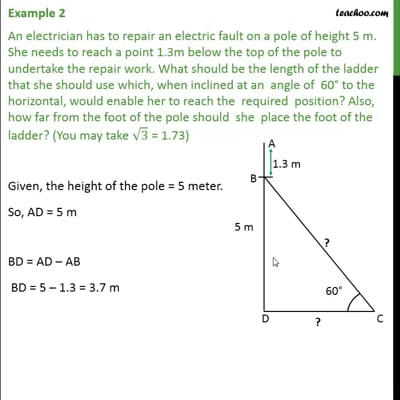Examples

Chapter 9 Class 10 Some Applications of Trigonometry (Term 2)
Serial order wiseThis video is only available for Teachoo black users

### Transcript

Example 2 An electrician has to repair an electric fault on a pole of height 5 m. She needs to reach a point 1.3m below the top of the pole to undertake the repair work. What should be the length of the ladder that she should use which, when inclined at an angle of 60° to the horizontal, would enable her to reach the required position? Also, how far from the foot of the pole should she place the foot of the ladder? (You may take √3 = 1.73) Given, the height of the pole = 5 meter. So, AD = 5 m BD = AD – AB BD = 5 – 1.3 = 3.7 m Here, we have to find the length of ladder, i.e. BC & distance from foot of the ladder to the foot of the pole, i.e. DC Finding length of ladder Since pole is vertical to ground ∠BDC = 90° Finding distance from foot of pole to foot of ladder In right angle triangle BCD, tan C = (𝑆𝑖𝑑𝑒 𝑜𝑝𝑝𝑜𝑠𝑖𝑡𝑒 𝑡𝑜 𝑎𝑛𝑔𝑙𝑒" " 𝐶)/(𝑆𝑖𝑑𝑒 𝑎𝑑𝑗𝑎𝑐𝑒𝑛𝑡 𝑡𝑜 𝑎𝑛𝑔𝑙𝑒" " 𝐶) tan 60° = 𝐵𝐷/𝐶𝐷 √3 = 𝐵𝐷/𝐶𝐷 √3 = 3.7/𝐶𝐷 CD = 3.7/√3 CD = 3.7/1.73 CD = 2.14m Hence, Distance from foot of pole to foot of ladder = CD = 2.14m